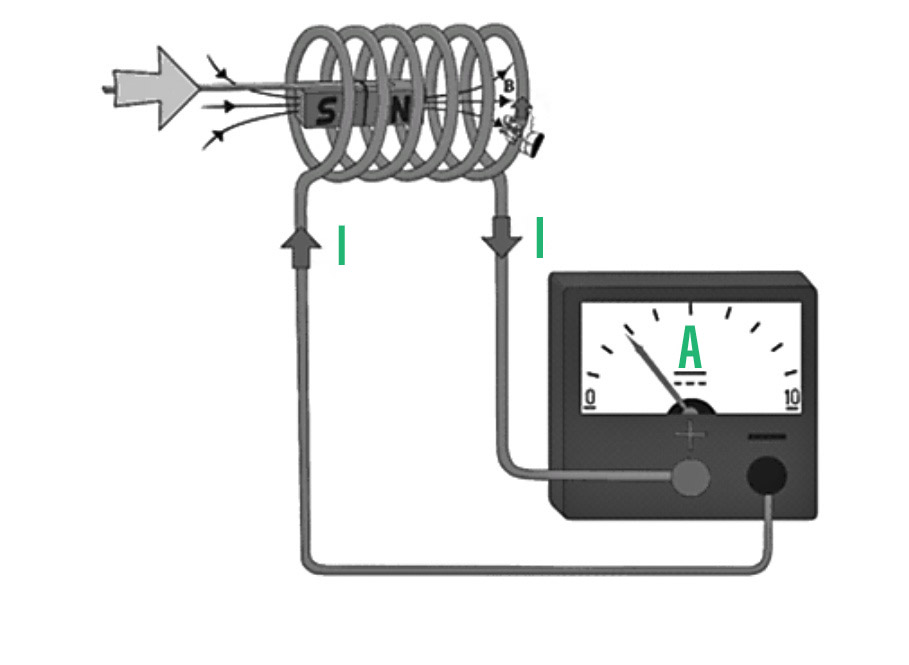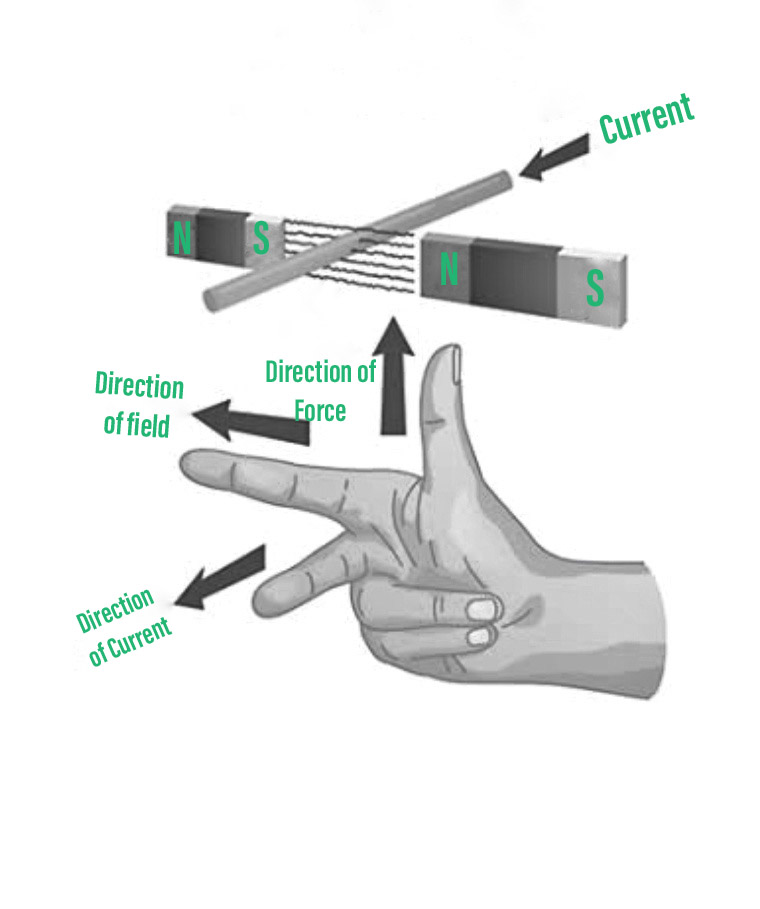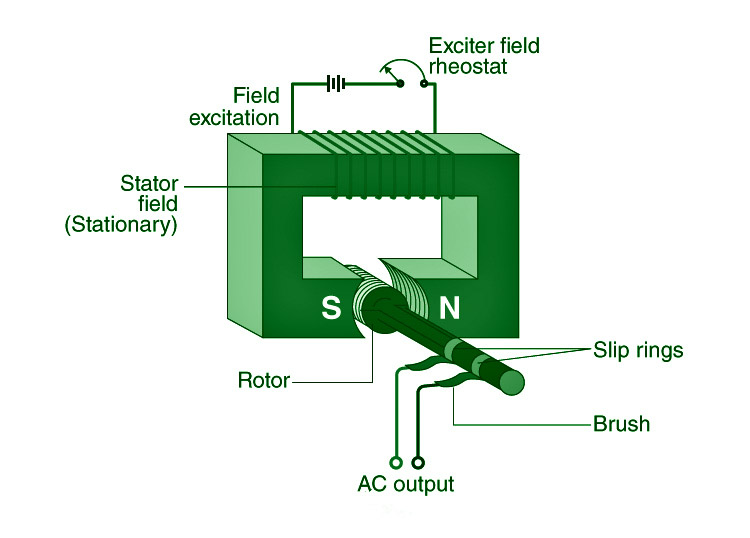# AC Generator – Principle, Construction, Working, Applications

• Last Updated : 02 Dec, 2021

A changing magnetic flux produces a voltage or current in a conductor, which is known as electromagnetic induction. It can happen when a solenoid’s magnetic flux is changed by moving a magnet.

There will be no generated voltage (electrostatic potential difference) across an electrical wire if the magnet is immobile. According to Michael Faraday, if the magnetic field is changing and (maintaining) movement while continually directing in the opposite direction (shifting its direction regularly), it would produce a voltage (thus the flow of alternating current).

### Electric Generator

A generator is a mechanical device that converts mechanical energy into electrical energy. The electricity generated at various power plants is produced by the generators installed there. When a coil spins in a magnetic field or moves relative to a magnet, it generates an electromotive force (emf) or potential difference.

Mechanical energy is required to rotate the coil, which is subsequently converted into electrical energy. The induced emf controls the flow of induced current via the coil, which is subsequently routed to our houses and used by us. The emf is caused by a phenomenon known as electromagnetic induction.

### Principle of Electric Generator

The operation of an electric generator is based on the electromagnetic induction idea. The phenomenon of electromagnetic induction refers to the generation of electric current in a circuit by varying the magnetic flux connected to the circuit.The total number of magnetic field lines passing through a particular region is known as magnetic flux. Moving a coil relative to a magnet alters the magnetic flux associated with the coil, resulting in emf in the coil.

Faraday established two laws that govern electromagnetic induction. These are their names:

1. When the amount of magnetic flux linked to a circuit changes, an emf is formed. The induced emf persists for as long as the magnetic flux varies.
2. In a circuit, the amplitude of induced emf is exactly proportional to the rate of change of magnetic flux coupled with the circuit.

There are several approaches for creating emf in a coil, including:

• Because of a coil’s and a magnet’s relative velocity.
• As a result of the relative motion of a coil and a current-carrying wire
• By changing the current in a conductor close to the coil.

Fleming’s Right Hand Rule may be used to identify the direction of the induced current produced in the coil: “Stretch the thumb, first finger, and centre finger of your right hand such that they are perpendicular to each other. The first finger points in the direction of the magnetic field, the thumb in the direction of conductor velocity, and the middle finger in the direction of induced current.”The coil of the electric generator is spun in a magnetic field to generate induced current. The resultant induced current fluctuates in amplitude and direction at a rate of thousands of times per second. Alternating current is the name given to this sort of energy (AC).

Direct current DC is used when the current produced by the electric generator does not change in direction or quantity. Depending on the type of current produced by the electric generator, we have a variety of generators.

Types of Electric Generator: Electric generators generate both alternating current (AC) and direct current (DC). Electric generators are classed as follows based on this:

### AC Generator

A machine that transforms mechanical energy into electrical energy is known as an AC generator. Mechanical energy is supplied to the AC Generator through steam turbines, gas turbines, and combustion engines. Alternating electrical power in the form of alternating voltage and current is the output.

Principle of AC Generator

AC generators function on Faraday’s law of electromagnetic induction states that electromotive force (EMF or voltage) is created in a current-carrying wire that cuts a uniform magnetic field. Rotating a conducting coil in a static magnetic field or rotating the magnetic field enclosing the stationary conductor can both be used to accomplish this. Because it is easier to extract induced alternating current from a stationary armature coil than from a revolving coil.

The EMF generated is determined by the number of armature coil turns, magnetic field intensity, and rotating field speed.

### Construction of AC GeneratorConstruction of AC Generator

The roles of each of these AC generator components are listed below.

1. Field- The field is made up of conductor coils that receive electricity from the source and generate magnetic flux. The armature is cut by the magnetic flux in the field, which produces a voltage. This voltage is the AC generator’s output voltage.
2. Armature- The portion of an AC generator that produces voltage is known as the armature. This component largely comprises of wire coils large enough to handle the generator’s full-load current.
3. Prime Mover- The prime mover is the component that drives the AC generator. A diesel engine, a steam turbine, or a motor might all be used as the prime mover.
4. Rotor- The rotor is the rotating component of the generator. The rotor is driven by the generator’s prime mover.
5. Stator- An AC generator’s stator is the stationary component. To reduce eddy current losses, the stator core is made up of a lamination of steel alloys or magnetic iron.
6. Slip Rings- Slip rings are electrical connectors that transport electricity from and to an AC generator’s rotor. They are primarily used to transfer electricity from a fixed device to a revolving one.

### Working of an AC Generator

The flux linkage of the armature varies continually as it revolves between the poles of the magnet on an axis perpendicular to the magnetic field. An electric current travels through the galvanometer, slip rings and brushes as a consequence. The galvanometer changes its value from positive to negative. This implies that the galvanometer is receiving an alternating current. Fleming’s Right-Hand Rule can be used to determine the direction of the induced current.

Direct Current Generator (DC Generator): The current produced by this form of electric generator does not alter direction or amplitude. As a result, the frequency of DC is always zero.

### Advantages of AC Generators over DC Generators

• Through transformers, AC generators may be simply stepped up and down.
• Because of the step-up functionality, the transmission link size in AC Generators is less.
• The losses in AC generators are lower than in DC machines.
• An AC generator is much smaller than a DC generator.

### Sample Question

Question 1: What are the advantages of alternating current versus direct current?

The benefits of AC over DC are as follows:

• With the aid of a transformer, AC may be obtained at any required voltage.
• Less electricity is wasted during transmission when utilising AC.
• AC machines are strong and long-lasting, requiring little maintenance.

Question 2: What is the operating principle of an electric generator?

The electromagnetic induction principle governs the operation of an electric generator.

Question 3: What is a commutator?

It is a device that links the armature of a DC generator to the external circuit and assists in maintaining the current direction in the external circuit. Every half-turn, it switches the connection of the armature ends to the ends of the external circuit.

Question 4: What is electromagnetic induction?

The phenomenon of electromagnetic induction occurs when current is generated in a circuit by altering the magnetic flux associated with it.

Question 5: What is an electric generator?

A generator is a machine that transforms mechanical energy into electrical energy.

Question 6: Which rule is used to determine the direction of induced current in an AC generator?